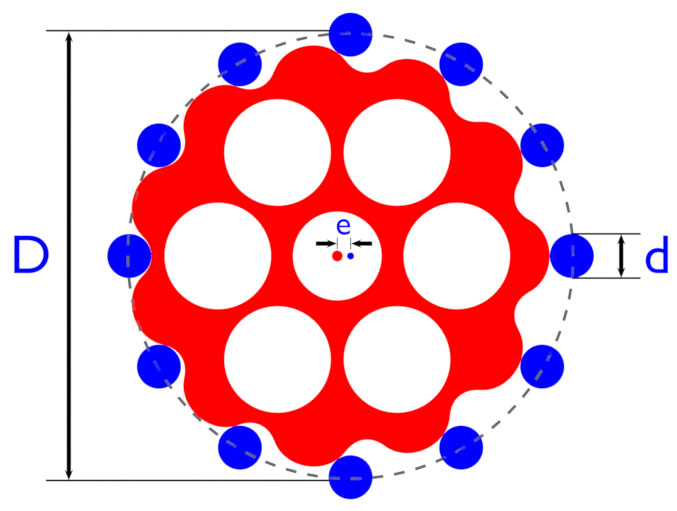Home  •  Tutorials  •  Calculators  •  3D Models  •  About  •  Contact

Hypocycloid Gear Calculator

The following online calculator computes the parametric equations of the cycloid disk of a hypocycloid drive.A hypocycloid drive is defined by just four easy-to-understand parameters:

• D - Diameter of the ring on which the centers of the pins are positioned;
• d - Diameter of the pins themselves (shown in blue);
• N - Number of pins;
• e - Eccentricity, or the shift of the cycloid disk's center relative to the center of the pin ring.

The parametric equations generated by this calculator define an epitrochoid curve from which the actual profile of the cycloid disk (shown in red) is easily obtained using Blender's Inset tool. The inset amount equals the pin radius (d / 2).

Basic Dimensions
ItemSymbolValue
Ring DiameterD
Pin Diameterd
Number of PinsN
Eccentricitye
Inset Amount (d / 2)i
Angle between pins (360° / N)ε

 X equation Y equation Z equation U Min, Max, Step